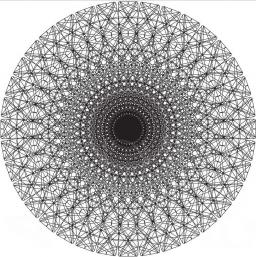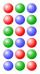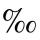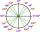# Comparing powers

How many times is number 56 larger than number 46?

Correct result:

x =  3.81

#### Solution:

$x=\frac{{5}^{6}}{{4}^{6}}={\left(\frac{5}{4}\right)}^{6}=3.81$We would be pleased if you find an error in the word problem, spelling mistakes, or inaccuracies and send it to us. Thank you!## Next similar math problems:

• TransformerTransformer - U1 = 230 V, N1 = 300, N2 = 1,200, I1 = 4 A. Calculate the transformation ratio, voltage and current in the secondary coil.
• Functions f,gFind g(1) if g(x) = 3x - x2 Find f(5) if f(x) = x + 1/2
• First manWhat is the likelihood of a random event where are five men and seven women first will leave the man?
• Percentage increaseIncrease number 400 by 3.5%
• Two equationsSolve equations (use adding and subtracting of linear equations): -4x+11y=5 6x-11y=-5
• Nineteenth memberFind the nineteenth member of the arithmetic sequence: a1=33 d=5 find a19
• 6 termsFind the first six terms of the sequence. a1 = 7, an = an-1 + 6
• TreesA certain species of tree grows an average of 0.5 cm per week. Write an equation for the sequence that represents the weekly height of this tree in centimeters if the measurements begin when the tree is 200 centimeters tall.
• Percent to numberTo increase that number by 5 percent, we need to multiply it by:
• One halfOne half of ? is: ?
• PermillHow many permill is 978 from 84370?
• PowersExpress the expression ? as the n-th power of the base 10.What is greater angle? -0.7 radians or -0.7π radians?The factory consists of three auxiliary divisions total 2,406 employees. The second division has 76 employees less than 1st division and 3rd division has 212 employees more than the 2nd. How many employees has each division?The difference between the two numbers is 74. If we divide a larger number by a smaller one, we get a quotient 7 and the rest of 2. Determine both numbers.Number ?. Find the value of x.Which of the numbers 710, 107 is greater?